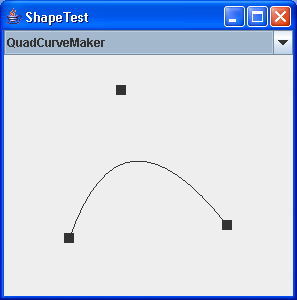# RectListManager is a class to manage a list of rectangular regions. : Shape « 2D Graphics GUI « Java

RectListManager is a class to manage a list of rectangular regions.

```
/*

Licensed to the Apache Software Foundation (ASF) under one or more
contributor license agreements.  See the NOTICE file distributed with
The ASF licenses this file to You under the Apache License, Version 2.0
(the "License"); you may not use this file except in compliance with

Unless required by applicable law or agreed to in writing, software
WITHOUT WARRANTIES OR CONDITIONS OF ANY KIND, either express or implied.
See the License for the specific language governing permissions and

*/

import java.awt.Rectangle;
import java.io.Serializable;
import java.util.Arrays;
import java.util.Collection;
import java.util.Comparator;
import java.util.Iterator;
import java.util.ListIterator;
import java.util.NoSuchElementException;

/**
* RectListManager is a class to manage a list of rectangular regions.
* This class contains methods to add new rectangles to the List, to
* merge rectangles in the list (based on a cost function), and
* functions to subract one RectListManager from another.  The main
* purpose of this class is to manage dirty regions on a display (for
* this reason it uses Rectangle not Rectangle2D).
*
* @author <a href="mailto:deweese@apache.org">Thomas DeWeese</a>
* @version \$Id: RectListManager.java 501844 2007-01-31 13:54:05Z dvholten \$
*/
public class RectListManager implements Collection {
Rectangle [] rects = null;
int size = 0;

Rectangle bounds = null;

public void dump() {
System.err.println("RLM: " + this + " Sz: " + size);
System.err.println("Bounds: " + getBounds());
for (int i=0; i<size; i++) {
Rectangle r = rects[i];
System.err.println("  [" + r.x + ", " + r.y + ", " +
r.width + ", " + r.height + ']' );
}
}

/**
* The comparator used to sort the elements of this List.
* Sorts on x value of Rectangle.
*/
public static Comparator comparator = new RectXComparator();

/**
* Construct a <tt>RectListManager</tt> from a Collection of Rectangles
* @param rects Collection that must only contain rectangles.
*/
public RectListManager(Collection rects) {
this.rects = new Rectangle[rects.size()];
Iterator i = rects.iterator();
int j=0;
while (i.hasNext())          // todo can be replaced by rects.toArray()
this.rects[j++] = (Rectangle)i.next();
this.size  = this.rects.length;

Arrays.sort(this.rects, comparator);
}

/**
* Construct a <tt>RectListManager</tt> from an Array of
* <tt>Rectangles</tt>
* @param rects Array of <tt>Rectangles</tt>, must not contain
*              any null entries.
*/
public RectListManager(Rectangle [] rects) {
this(rects, 0, rects.length);
}

/**
* Construct a <tt>RectListManager</tt> from an Array of
* <tt>Rectangles</tt>
* @param rects Array of <tt>Rectangles</tt>, must not contain
*              any null entries in the range [off, off+sz-1].
* @param off   The offset to start copying from in rects.
* @param sz    The number of entries to copy from rects.
*/
public RectListManager(Rectangle [] rects, int off, int sz) {
this.size  = sz;
this.rects = new Rectangle[sz];
System.arraycopy(rects, off, this.rects, 0, sz);
Arrays.sort(this.rects, comparator);
}

/**
* Construct a <tt>RectListManager</tt> from another
* <tt>RectListManager</tt> (data is copied).
* @param rlm RectListManager to copy.
*/
public RectListManager(RectListManager rlm) {
this(rlm.rects);
}

/**
* Construct a <tt>RectListManager</tt> with one rectangle
* @param rect The rectangle to put in this rlm.
*/
public RectListManager(Rectangle rect) {
this();
}

/**
* Construct an initially empty <tt>RectListManager</tt>.
*/
public RectListManager() {
this.rects = new Rectangle;
size = 0;
}

/**
* Construct an initially empty <tt>RectListManager</tt>,
* with initial <tt>capacity</tt>.
* @param capacity The inital capacity for the list.  Setting
*                 this appropriately can save reallocations.
*/
public RectListManager(int capacity) {
this.rects = new Rectangle[capacity];
}

public Rectangle getBounds() {
if (bounds != null )
return bounds;
if (size == 0) return null;
bounds = new Rectangle(rects);
for (int i=1; i< size; i++) {
Rectangle r = rects[i];
if (r.x < bounds.x) {
bounds.width = bounds.x+bounds.width-r.x;
bounds.x = r.x;
}
if (r.y < bounds.y) {
bounds.height = bounds.y+bounds.height-r.y;
bounds.y = r.y;
}
if (r.x+r.width > bounds.x+bounds.width)
bounds.width = r.x+r.width-bounds.x;
if (r.y+r.height > bounds.y+bounds.height)
bounds.height = r.y+r.height-bounds.y;
}
return bounds;
}

/**
* Standard <tt>Object</tt> clone method.
*/
public Object clone() throws CloneNotSupportedException {
return copy();
}

/**
* Similar to clone only strongly typed
*/
public RectListManager copy() {
return new RectListManager(rects);
}

/**
* Returns the number of elements currently stored in this collection.
*/
public int size() { return size; }

/**
* Returns true if this collection contains no elements.
*/
public boolean isEmpty() { return (size==0); }

public void clear() {
Arrays.fill( rects, null );
size=0;
bounds = null;
}

/**
* Returns an iterator over the elements in this collection
*/
public Iterator iterator() {
return new RLMIterator();
}

/**
* Returns a list iterator of the elements in this list
* (in proper sequence).
*/
public ListIterator listIterator() {
return new RLMIterator();
}

public Object [] toArray() {
Object [] ret = new Rectangle[size];
System.arraycopy(rects, 0, ret, 0, size);
return ret;
}

/**
* fill the given array a with values from my internal <code>rects</code>.
* when a is not large enough, a new array is allocated, filled and returned.
* the method works only, when a is a Object[] or a Rectange[].
* When this is not the case, the a[] is just cleared.
*
* @param a array to fill (must not be null!)
* @return the content of rects, either in a[] or a fresh array.
*/
public Object [] toArray(Object[] a) {
Class t = a.getClass().getComponentType();
if ((t != Object.class) &&
(t != Rectangle.class)) {
// Nothing here for it...
Arrays.fill( a, null );
return a;
}

if (a.length < size)
a = new Rectangle[size];
System.arraycopy(rects, 0, a, 0, size);
Arrays.fill( a, size, a.length, null );

return a;
}

return true;
}

/**
* Ensures that this collection contains the specified element
* @param rect The rectangle to add
*/
}

/**
* Ensures that this collection contains the specified element
* l is the lower bound index for insertion r is upper
* bound index for insertion.
* @param rect The rectangle to add
* @param l the lowest possible index for a rect with
*          greater 'x' coord.
* @param r the highest possible index for a rect with
*          greater 'x' coord.
*/
protected void add(Rectangle rect, int l, int r) {
ensureCapacity(size+1);
int idx=l;
while (l <= r) {
idx = (l+r)/2;
while ((rects[idx] == null) && (idx <r)) idx++;
if (rects[idx] == null) {
// All 'null' from center to r so skip them
r = (l+r)/2;
idx = (l+r)/2;
if (l>r)
idx=l;
while ((rects[idx] == null) && (idx > l)) idx--;
if (rects[idx] == null) {
rects[idx] = rect;
return;
}
}
if (rect.x == rects[idx].x) break;
if (rect.x <  rects[idx].x) {
if (idx == 0) break;
if ((rects[idx-1] != null) &&
(rect.x >= rects[idx-1].x)) break;
r = idx-1;
} else {
if (idx == size-1)  {idx++; break; }
if ((rects[idx+1] != null) &&
(rect.x <= rects[idx+1].x)) { idx++; break;}
l = idx+1;
}
}

if (idx < size) {
System.arraycopy(rects, idx,
rects, idx+1, size-idx);
}

// if (idx!=0) System.out.print(rects[idx-1].x);
// else System.out.print("[First]");
// System.out.print(" " + rect.x + " ");
// if (idx<size) System.out.print(rects[idx+1].x);
// else System.out.print("[last]");
// System.out.println("");

rects[idx] = rect;
size++;
bounds=null;
}

if (c instanceof RectListManager) {
} else {
}

return (c.size() != 0);
}

public boolean contains(Object o) {
Rectangle rect = (Rectangle)o;
int l=0, r=size-1, idx=0;
while (l <= r) {
idx = (l+r) >>> 1;
if (rect.x == rects[idx].x) break;
if (rect.x <  rects[idx].x) {
if (idx == 0) break;
if (rect.x >= rects[idx-1].x) break;
r = idx-1;
} else {
if (idx == size-1)  {idx++; break; }
if (rect.x <= rects[idx+1].x) { idx++; break;}
l = idx+1;
}
}
// Didn't find any rect with the same x value.
if (rects[idx].x != rect.x) return false;

// Search towards 0 from idx for rect that matches
for (int i=idx; i>=0; i--){
if (rects[idx].equals(rect)) return true;
if (rects[idx].x != rect.x)  break;
}

// Search towards size from idx for rect that matches
for (int i=idx+1; i<size; i++) {
if (rects[idx].equals(rect)) return true;
if (rects[idx].x != rect.x)  break;
}

// No match...
return false;
}

/**
* Returns true if this collection contains all of the elements in
* the specified collection.
*/
public boolean containsAll(Collection c) {
if (c instanceof RectListManager)
return containsAll((RectListManager)c);
return containsAll(new RectListManager(c));
}

public boolean containsAll(RectListManager rlm) {
int x, xChange = 0;
for (int j=0, i=0; j<rlm.size; j++) {
i=xChange;
while(rects[i].x < rlm.rects[j].x) {
i++;
if (i == size) return false;
}
xChange = i;
x = rects[i].x;
while (!rlm.rects[j].equals(rects[i])) {
i++;
if (i == size) return false; // out of rects
if (x != rects[i].x)
return false; // out of the zone.
}
}
return true;
}

/**
* Removes a single instance of the specified element from this
* collection, if it is present.
* @param o Object to remove an matching instance of.
*/
public boolean remove(Object o) {
return remove((Rectangle)o);
}

/**
* Removes a single instance of the specified Rectangle from this
* collection, if it is present.
* @param rect Rectangle to remove an matching instance of.
*/
public boolean remove(Rectangle rect) {
int l=0, r=size-1, idx=0;
while (l <= r) {
idx = (l+r) >>> 1;
if (rect.x == rects[idx].x) break;
if (rect.x <  rects[idx].x) {
if (idx == 0) break;
if (rect.x >= rects[idx-1].x) break;
r = idx-1;
} else {
if (idx == size-1)  {idx++; break; }
if (rect.x <= rects[idx+1].x) { idx++; break;}
l = idx+1;
}
}
// Didn't find any rect with the same x value.
if (rects[idx].x != rect.x) return false;

// Search towards 0 from idx for rect that matches
for (int i=idx; i>=0; i--){
if (rects[idx].equals(rect)) {
System.arraycopy(rects, idx+1, rects, idx, size-idx);
size--;
bounds = null;
return true;
}
if (rects[idx].x != rect.x)  break;
}

// Search towards size from idx for rect that matches
for (int i=idx+1; i<size; i++) {
if (rects[idx].equals(rect)) {
System.arraycopy(rects, idx+1, rects, idx, size-idx);
size--;
bounds = null;
return true;
}
if (rects[idx].x != rect.x)  break;
}

// No match...
return false;
}

public boolean removeAll(Collection c) {
if (c instanceof RectListManager)
return removeAll((RectListManager)c);
return removeAll(new RectListManager(c));
}

public boolean removeAll(RectListManager rlm) {
int x, xChange = 0;
boolean ret = false;
for (int j=0, i=0; j<rlm.size; j++) {
i=xChange;
while ((rects[i] == null) ||
(rects[i].x < rlm.rects[j].x)) {
i++;
if (i == size) break;
}

if (i == size) break;

xChange = i;
x = rects[i].x;
while (true) {
if (rects[i] == null) {
i++;
if (i == size) break; // out of rects
continue;
}
if (rlm.rects[j].equals(rects[i])) {
rects[i] = null;
ret = true;
}
i++;
if (i == size)       break; // out of rects
if (x != rects[i].x) break; // out of the zone.
}
}

// Now we will go through collapsing the nulled entries.
if (ret) {
int j=0, i=0;
while (i<size) {
if (rects[i] != null)
rects[j++] = rects[i];
i++;
}
size = j;
bounds = null;
}
return ret;
}

public boolean retainAll(Collection c) {
if (c instanceof RectListManager)
return retainAll((RectListManager)c);
return retainAll(new RectListManager(c));
}
public boolean retainAll(RectListManager rlm) {
int x, xChange = 0;
boolean ret = false;

for (int j=0, i=0; j<size; j++) {
i=xChange;
while (rlm.rects[i].x < rects[j].x) {
i++;
if (i == rlm.size) break;
}
if (i == rlm.size) {
ret = true;
// No more rects will match anything from rlm
// so remove them from this RLM.
for (int k=j; k<size; k++)
rects[k] = null;
size = j;
break;
}

xChange = i;
x = rlm.rects[i].x;
while (true) {
if (rects[j].equals(rlm.rects[i])) break;
i++;
if ((i == rlm.size) ||
(x != rlm.rects[i].x)) {
// Out of zone or rects
rects[j] = null;
ret = true;
break;
}
}
}

// Now we will go through collapsing the nulled entries.
if (ret) {
int j=0, i=0;
while (i<size) {
if (rects[i] != null)
rects[j++] = rects[i];
i++;
}
size = j;
bounds = null;
}
return ret;
}

/**
* Adds the contents of <tt>rlm</tt> to this RectListManager.  No
* collapsing of rectangles is done here the contents are simply
* added (you should generally call 'mergeRects' some time after
* this operation before using the contents of this
* RectListManager.
* @param rlm The RectListManager to add the contents of.  */
if (rlm.size == 0)
return;

Rectangle [] dst = rects;
if (rects.length < (size+rlm.size)) {
dst = new Rectangle[size+rlm.size];
}

if (size == 0) {
System.arraycopy(rlm.rects, 0, dst, size, rlm.size);
size = rlm.size;
bounds = null;
return;
}

Rectangle [] src1   = rlm.rects;
int          src1Sz = rlm.size;
int          src1I  = src1Sz-1;

Rectangle [] src2   = rects;
int          src2Sz = size;
int          src2I  = src2Sz-1;

int dstI = size+rlm.size-1;
int x1 = src1[src1I].x;
int x2 = src2[src2I].x;

while (dstI >= 0) {
if (x1 <= x2) {
dst[dstI] = src2[src2I];
if (src2I == 0) {
System.arraycopy(src1, 0, dst, 0, src1I+1);
break;
}
src2I--;
x2 = src2[src2I].x;
} else {
dst[dstI] = src1[src1I];
if (src1I == 0) {
System.arraycopy(src2, 0, dst, 0, src2I+1);
break;
}
src1I--;
x1 = src1[src1I].x;
}
dstI--;
}
rects = dst;
size += rlm.size;
bounds = null;
}

if (size == 0) return;
Rectangle r, cr, mr;
int cost1, cost2, cost3;
mr = new Rectangle();
Rectangle []splits = new Rectangle;
for (int j, i=0; i<size; i++) {
r = rects[i];
if (r == null) continue;
(r.height*r.width));
do {
for (j=i+1; j<size; j++) {
cr = rects[j];
if ((cr == null) || (cr == r)) continue;
if (cr.x >= maxX) {
// No more merges can happen.
j = size;
break;
}
(cr.height*cr.width));

mr = r.union(cr);
(mr.height*mr.width));
if (cost3 <= cost1+cost2) {
r = rects[i] = mr;
rects[j] = null;
cost1 = cost3;
j=-1;
break;
}

if (!r.intersects(cr)) continue;

splitRect(cr, r, splits);
int splitCost=0;
int l=0;
for (int k=0; k<4; k++) {
if (splits[k] != null) {
Rectangle sr = splits[k];
// Collapse null entries in first three
// (That share common 'x').
if (k<3) splits[l++] = sr;
(sr.height*sr.width));
}
}
if (splitCost >= cost2) continue;

// Insert the splits.
if (l == 0) {
// only third split may be left (no common 'x').
rects[j] = null;
if (splits != null)
continue;
}

rects[j] = splits;
if (l > 1)
insertRects(splits, 1, j+1, l-1);
if (splits != null)
}

// if we merged it with another rect then
// we need to check all the rects up to i again,
// against the merged rect.
} while (j != size);
}

// Now we will go through collapsing the nulled entries.
int j=0, i=0;
float area=0;
while (i<size) {
if (rects[i] != null) {
r = rects[i];
rects[j++] = r;
(r.height*r.width);
}
i++;
}
size = j;
bounds=null;
r = getBounds();
if (r == null) return;
rects = r;
size=1;
}
}

Rectangle r, sr;
int cost;
int jMin=0;
Rectangle [] splits = new Rectangle;

for(int i=0; i<size; i++) {
r = rects[i]; // Canidate rect...
(r.height*r.width));
for (int j=jMin; j<rlm.size; j++) {
sr = rlm.rects[j]; // subtraction rect.

// Check if the canidate rect starts after
// the end of this rect in 'x' if so
// go to the next one.
if (sr.x+sr.width < r.x) {
// If this was jMin then increment jMin (no
// future canidate rect will intersect this rect).
if (j == jMin) jMin++;
continue;
}

// Check if the rest of the rects from rlm are past
// the end of the canidate rect.  If so we are
// done with this canidate rect.
if (sr.x > r.x+r.width)
break;

// If they don't insersect then go to next sub rect.
if (!r.intersects(sr))
continue;

// Now we know they intersect one another lets
// figure out how...

splitRect(r, sr, splits);

int splitCost=0;
Rectangle tmpR;
for (int k=0; k<4; k++) {
tmpR = splits[k];
if (tmpR != null)
(tmpR.height*tmpR.width));
}

if (splitCost >= cost)
// This isn't ideal as depending on the order
// Stuff is done in we might later kill some of
// these rectangles (hence lowering the cost).
// For this reason it is probably best of the
// subtract list has been merged as this will help
// reduce the instances where this will happen.
continue;

// Collapse null entries in first three elements
// split 0, 1, 2 (entries that share a common 'x').
int l = 0;
for (int k=0; k<3; k++) {
if (splits[k] != null)
splits[l++] = splits[k];
}

// Fully covered (or only split 3 survived which we
// will visit later) this canidate rect goes away.
if (l==0) {
rects[i].width = 0;
// Insert the third split (if any) at the
// proper place in rects list.
if (splits != null)
break;
}

// Otherwise replace the canidate with the top of
// the split, since it only shrunk it didn't grow,
// we know that the previous subtract rects don't
// intersect it.
r        = splits;
rects[i] = r;
(r.height*r.width));

// Add the remainder of the rects that
// share 'r.x' (if any).  Possible
// are split 1, and split 2.
if (l > 1)
insertRects(splits, 1, i+1, l-1);

// Insert the third split (if any) at the
// proper place in rects list.
if (splits != null)
}
}

// Now we will go through collapsing the nulled entries.
int j=0, i=0;
while (i<size) {
if (rects[i].width == 0)
rects[i] = null;
else
rects[j++] = rects[i];
i++;
}
size = j;
bounds = null;
}

protected void splitRect(Rectangle r, Rectangle sr,
Rectangle []splits) {
// We split the canidate rectrect into four parts.  In
// many cases one or more of these will be empty.
//
//    +-------------------------------------+ ry0
//    |                                     |
//    |                                     |
//    |          Split 0                    |
//    |                                     |
//    |                                     |
// ------------+-----------------+--------------- sry0
//    |        |                 |          |
//    | Split2 |   subtracted    | Split 3  |
//    |        |   rect          |          |
//    |        |                 |          |
// ------------+-----------------+--------------- sry1
//    |       srx0              srx1        |
//    |                                     |
//    |          Split 1                    |
//    |                                     |
//    +-------------------------------------+ ry1
//   rx0                                   rx1

int rx0 = r.x;
int rx1 = rx0+r.width-1;
int ry0 = r.y;
int ry1 = ry0+r.height-1;

int srx0 = sr.x;
int srx1 = srx0+sr.width-1;
int sry0 = sr.y;
int sry1 = sry0+sr.height-1;

if ((ry0 < sry0) && (ry1 >= sry0)) {
splits = new Rectangle(rx0, ry0, r.width, sry0-ry0);
ry0 = sry0;
} else {
splits = null;
}

if ((ry0 <= sry1) && (ry1 > sry1)) {
splits = new Rectangle(rx0, sry1+1, r.width, ry1-sry1);
ry1 = sry1;
} else {
splits = null;
}

if ((rx0 < srx0) && (rx1 >= srx0)) {
splits = new Rectangle(rx0, ry0, srx0-rx0, ry1-ry0+1);
} else {
splits = null;
}

if ((rx0 <= srx1) && (rx1 > srx1)) {
splits= new Rectangle(srx1+1, ry0, rx1-srx1, ry1-ry0+1);
} else {
splits = null;
}
}

protected void insertRects(Rectangle[] rects, int srcPos,
int dstPos, int len) {
if (len == 0) return;

// Make sure we have room.
ensureCapacity(size+len);

// Move everything after pos up...
for (int i=size-1; i>=dstPos; i--)
this.rects[i+len] = this.rects[i];

// Put the new rects in.
System.arraycopy( rects, srcPos, this.rects, dstPos, len );

size += len;
}

public void ensureCapacity(int sz) {
if (sz <= rects.length)
return;
int nSz = rects.length + (rects.length>>1) + 1;
while (nSz < sz)
nSz+=(nSz>>1)+1;

Rectangle [] nRects = new Rectangle[nSz];
System.arraycopy(rects, 0, nRects, 0, size);

rects = nRects;
}

/**
* Comparator for ordering rects in X.
*
* Note: this comparator imposes orderings that are inconsistent
*       with equals.
*/
private static class RectXComparator implements Comparator, Serializable {

RectXComparator() { }

public final int compare(Object o1, Object o2) {
return ((Rectangle)o1).x-((Rectangle)o2).x;
}
}

private class RLMIterator implements ListIterator {
int idx = 0;
boolean removeOk = false;
boolean forward  = true;
RLMIterator() { }

public boolean hasNext() { return idx < size; }
public int nextIndex() { return idx; }
public Object next() {
if (idx >= size)
throw new NoSuchElementException("No Next Element");
forward = true;
removeOk = true;
return rects[idx++];
}

public boolean hasPrevious() { return idx > 0; }
public int previousIndex() { return idx-1; }
public Object previous() {
if (idx <= 0)
throw new NoSuchElementException("No Previous Element");
forward = false;
removeOk = true;
return rects[--idx];
}

public void remove() {
if (!removeOk)
throw new IllegalStateException
("remove can only be called directly after next/previous");

if (forward) idx--;
if (idx != size-1)
System.arraycopy(rects, idx+1, rects, idx, size-(idx+1));
size--;
rects[size] = null;
removeOk = false;
}

public void set(Object o) {
Rectangle r = (Rectangle)o;

if (!removeOk)
throw new IllegalStateException
("set can only be called directly after next/previous");

if (forward) idx--;

if (idx+1<size) {
if (rects[idx+1].x < r.x)
throw new UnsupportedOperationException
("RectListManager entries must be sorted");
}
if (idx>=0) {
if (rects[idx-1].x > r.x)
throw new UnsupportedOperationException
("RectListManager entries must be sorted");
}

rects[idx] = r;
removeOk = false;
}

Rectangle r = (Rectangle)o;
if (idx<size) {
if (rects[idx].x < r.x)
throw new UnsupportedOperationException
("RectListManager entries must be sorted");
}
if (idx!=0) {
if (rects[idx-1].x > r.x)
throw new UnsupportedOperationException
("RectListManager entries must be sorted");
}
ensureCapacity(size+1);
if (idx != size)
System.arraycopy(rects, idx, rects, idx+1, size-idx);
rects[idx] = r;
idx++;
removeOk = false;
}
}
}

```

### Related examples in the same category

 1 Creating Basic Shapes 2 fillRect (int, int, int, int) method draws a solid rectangle 3 Creating a Shape Using Lines and Curves 4 Combining Shapes 5 Draw rectangles, use the drawRect() method. To fill rectangles, use the fillRect() method 6 Draw line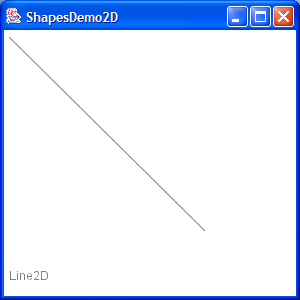7 Draw a Polygon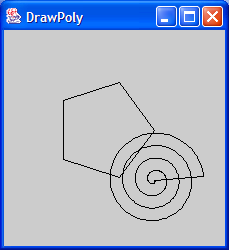8 Draw an oval outline 9 Draw a (Round)rectangle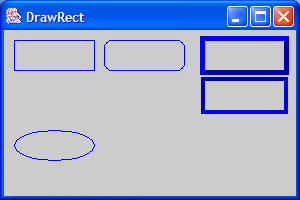10 Fill a polygon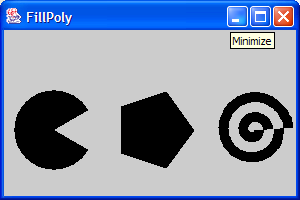11 Fill a solid oval 12 Fill a (Round)rectangle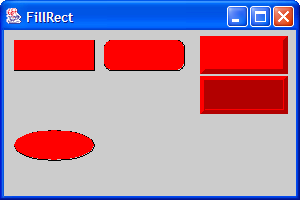13 Change font14 Draw rectangle 2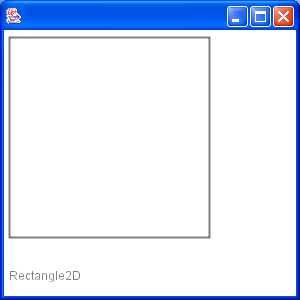15 Draw Arc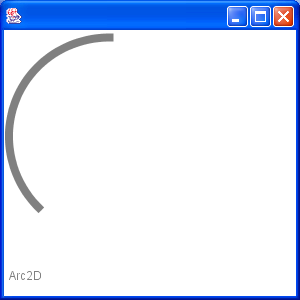16 Draw Ellipse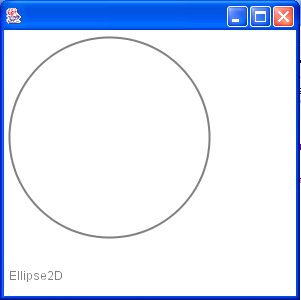17 Fill a Rectangle 2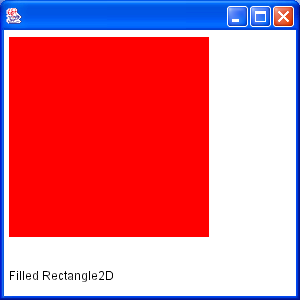18 Fill Arc 2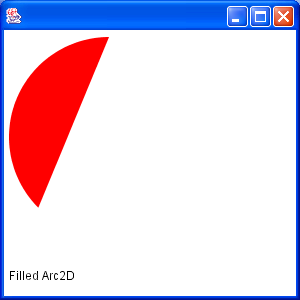19 Draw text20 Draw unicode string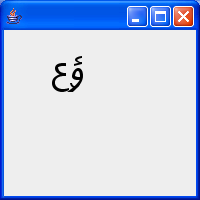21 Shape combine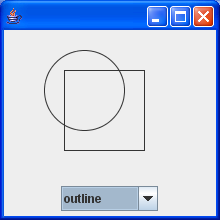22 Effects23 Mouse drag and drop to draw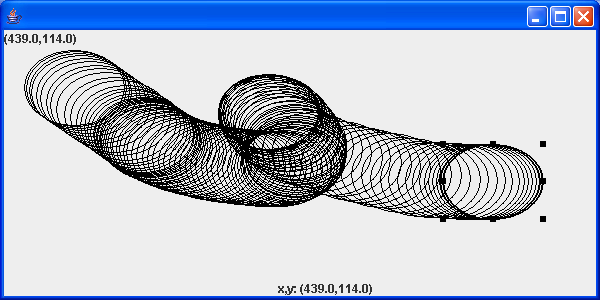24 Arc demonstration: scale, move, rotate, sheer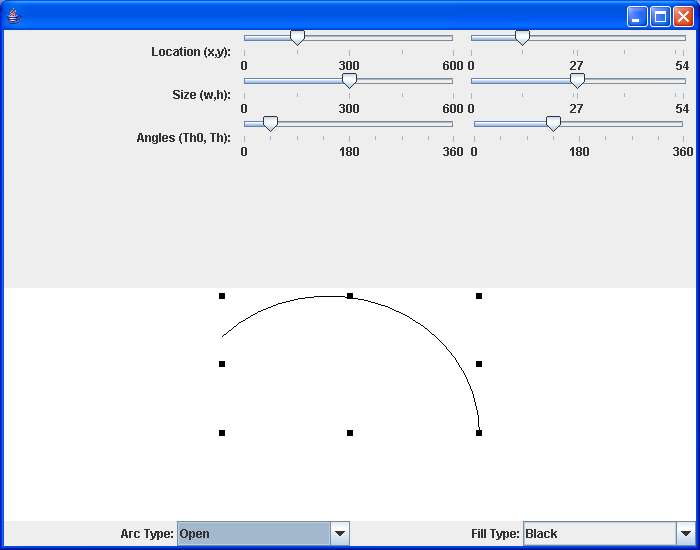25 Hypnosis Spiral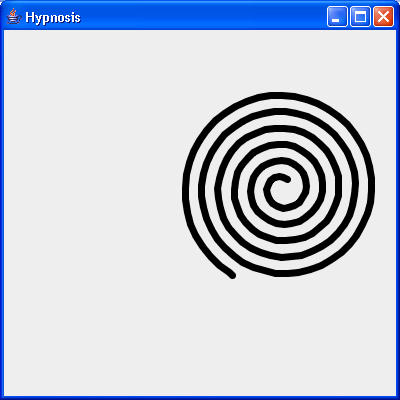26 GlyphVector.getNumGlyphs() 27 Resize a shape 28 Rectangle with rounded corners drawn using Java 2D Graphics API 29 Compares two ellipses and returns true if they are equal or both null. 30 Compares two lines are returns true if they are equal or both null. 31 Creates a diagonal cross shape. 32 Creates a diamond shape. 33 Creates a new Stroke-Object for the given type and with. 34 Creates a region surrounding a line segment by 'widening' the line segment. 35 Creates a triangle shape that points downwards. 36 Creates a triangle shape that points upwards. 37 Generate Polygon 38 Polygon with float coordinates. 39 Polyline 2D 40 Serialises a Shape object. 41 Tests two polygons for equality. If both are null this method returns true. 42 Union two rectangles 43 Calculate Intersection Clip 44 Draws a shape with the specified rotation about (x, y). 45 Checks, whether the given rectangle1 fully contains rectangle 2 (even if rectangle 2 has a height or width of zero!). 46 Reads a Point2D object that has been serialised by the writePoint2D(Point2D, ObjectOutputStream)} method. 47 Returns a point based on (x, y) but constrained to be within the bounds of a given rectangle. 48 Fill Rectangle2D.Double and Ellipse2D.Double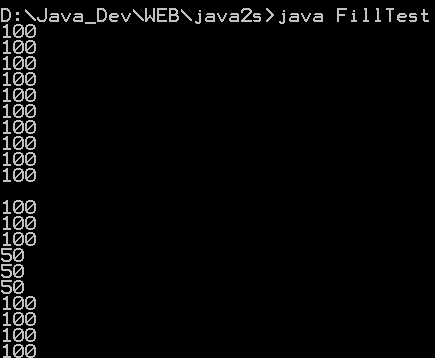49 This program demonstrates the various 2D shapes